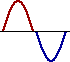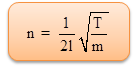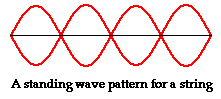Click to Chat

1800-1023-196

+91-120-4616500

CART 0

• 0

MY CART (5)

Use Coupon: CART20 and get 20% off on all online Study Material

ITEM
DETAILS
MRP
DISCOUNT
FINAL PRICE
Total Price: Rs.

There are no items in this cart.
Continue Shopping• Complete Physics Course - Class 11
• OFFERED PRICE: Rs. 2,968
• View Details

```Stationary WavesWhen two progressive waves of same amplitude and wavelength travelling along a straight line in opposite directions superimpose on each other, stationary waves are formed.

Analytical method

Let us consider a progressive wave of amplitude a and wavelength λ travelling in the direction of X axis.

y1 = a sin 2π [t/T – x/λ]         …... (1)

This wave is reflected from a free end and it travels in the negative direction of X axis, then

y2 = a sin 2π [t/T + x/λ]         …... (2)

According to principle of superposition, the resultant displacement is,y = y1+y2

= a [sin 2π (t/T – x/λ) + sin 2π (t/T + x/λ)]

= a [2sin (2πt/T) cos  (2πx/λ)]

So, y = 2a cos (2πx/λ) sin (2πt/T)         …... (3)

This is the equation of a stationary wave.

(a) At points where x = 0, λ/2, λ, 3λ/2, the values of cos 2πx/λ = ±1

∴ A = + 2a. At these points the resultant amplitude is maximum. They are called antinodes as shown in figure.

(b) At points where x = λ/4, 3λ/4, 5λ/4..... the values of cos 2πx/λ = 0.

∴ A = 0. The resultant amplitude is zero at these points. They are called nodes.The  distance  between  any  two successive antinodes or nodes is equal to λ/2 and the distance between an antinode and a node is λ/4.

(c) When t = 0, T/2, T, 3T/2, 2T,.... then sin 2πt/T = 0, the displacement is zero.

(d) When t = T/4, 3T/4, 5T/4 etc,....sin 2πt/T = ±1,  the displacement is maximum.

Characteristics of stationary waves

The waveform remains stationary.

Nodes and antinodes are formed alternately.

The points where displacement is zero are called nodes and the points where the displacement is maximum are called antinodes.

Pressure changes are maximum at nodes and minimum at antinodes.All the particles except those at the nodes, execute simple harmonic motions of same period.

Amplitude of each particle is not the same, it is maximum at antinodes decreases gradually and is zero at the nodes.

The velocity of the particles at the nodes is zero. It increases gradually and is maximum at the antinodes.

Distance between any two consecutive nodes or antinodes is equal to λ2 , whereas the distance between a node and its adjacent antinode is equal to λ/4 .

There is no transfer of energy. All the particles of the medium pass through their mean position simultaneously twice during each vibration.

Particles in the same segment vibrate in the same phase and between the neighbouring segments, the particles vibrate in opposite phase.

Standing waves in strings

In musical instruments like sitar, violin, etc. sound is produced due to the vibrations of the stretched strings. Here, we shall discuss the different modes of vibrations of a string which is rigidly fixed at both ends.

When a string under tension is set into vibration, a transverse progressive wave moves towards the end of the wire and gets reflected. Thus stationary waves are formed.

SonometerThe sonometer consists of a hollow sounding box about a metre long. One end of a thin metallic wire of uniform cross-section is fixed to a hook and the other end is passed over a pulley and attached to a weight hanger as shown in figure. The wire is stretched over two knife edges P and Q by adding sufficient weights on the hanger. The distance between the two knife edges can be adjusted to change the vibrating length of the wire.

A transverse stationary wave is set up in the wire. Since the ends are fixed, nodes are formed at P and Q and antinode is formed in the middle.

The length of the vibrating l = λ/2

Thus λ = 2l. If n is the frequency of the vibrating segment, then,

n = v/λ = v/2l                     …... (1)

We know that, v = √T/m where T is the tension and m is the mass per unit length of the wire.

Thus,…... (2)

Modes of vibration of stretched string(i) Fundamental frequency

If a wire is stretched between two points, a transverse wave travels along the wire and is reflected at the fixed end. A transverse stationary wave is thus formed as shown in figure.

When a wire AB of length l is made to vibrate in one segment then, l = λ1/2

λ1 = 2l. This gives the lowest frequency called fundamental frequency, n1 = v/λ1

So, n1 = (1/2l) √T/m                …... (3)

(ii) Overtones in stretched string

If the wire AB is made to vibrate in two segments then l = λ2/2 +  λ2/2

So, λ2 = l

But, n2 = v/λ2

So, n2 = 1/l √T/m = 2n1          …... (4)

n2 is the frequency of the first overtone.

Since the frequency is equal to twice the fundamental, it is also known as second harmonic.

Similarly, higher overtones are produced, if the wire vibrates with more segments. If there are P segments, the length of each segment is

l/p = λp/2

Or, λp = 2l/P

So, frequency, np = (P/2l)√T/m = Pn1          …... (5)

(i.e) Pth harmonic corresponds to (P–1)th overtone.

Refer this video to know more about standing wave

Laws of transverse vibrations of stretched strings

The laws of transverse vibrations of stretched strings are (i) the law of length (ii) law of tension and (iii) the law of mass.

(i) For a given wire (m is constant), when T is constant, the fundamental frequency of vibration is inversely proportional to the vibrating length (i.e)

n ∝ 1/l

Or, nl = constant

(ii) For constant l and m, the fundamental frequency is directly proportional to the square root of the tension (i.e)

n ∝ √T.

(iii) For constant l and T, the fundamental frequency varies inversely as the square root of the mass per unit length of the wire

(i.e) n ∝ 1/√m.The behavior of the waves at the points of minimum and maximum vibrations (nodes and antinodes) contributes to the constructive interference which forms the resonant standing waves.

The term standing wave is often applied to a resonant mode of an extended vibrating object.

The resonance is created by constructive interference of two waves which travel in opposite directions in the medium, but the visual effect is that of an entire system moving in simple harmonic motion.

Standing waves don't go anywhere, but they do have regions where the disturbance of the wave is quite small, almost zero.

Standing waves can form under a variety of conditions, but they are easily demonstrated in a medium which is finite or bounded.

In general, standing waves can be produced by any two identical waves traveling in opposite directions that have the right wavelength. In a bounded medium, standing waves occur when a wave with the correct wavelength meets its reflection.

Standing waves don't form under just any circumstances. They require that energy be fed into a system at an appropriate frequency.

Standing waves are always associated with resonance.

In non-electronic instruments, the stable, controlled vibration is produced by a standing wave.

A standing wave pattern is a vibrational pattern created within a medium when the vibrational frequency of the source causes reflected waves from one end of the medium to interfere with incident waves from the source.

Problem (JEE Main):

Equations of two progressive waves at a certain point in a medium are given by y1 = a sin (ωt + φ1) and y2 = a sin (ωt + φ2). If amplitude and time period of resultant wave formed by the superposition of these two waves is same as that of both the waves, then

φ1 – φ2 is,

(a) π/3                    (b) 2π/3

(c) π/6                    (d) π/4

Solution:

A2 = a12 + a22 + 2a1a2 cos φ

Here, φ =  φ1 – φ2, A = a1 = a2 = a

Substituting these values in equation (1), we get,

cos φ = – ½

Or, φ = 2π/3

Thus, from the above observation we conclude that, option (b) is correct.Question 1

A tube closed at one end and containing air, produces, when excited, the fundamental note of frequency 512 Hz. If the tube is open at both ends, the fundamental frequency that can be excited is (in Hz) (I.I.T. 86)

(a) 1024

(b) 512

(c) 256

(d) 128

Question 2

Velocity of sound in air is 320 m/s. a pipe closed at one end has length of 1 m. neglecting end corrections, the air column in the pipe can resonates for sound of frequency (I.I.T 89)

(a) 80 Hz

(b) 320 Hz

(c) 400 Hz

(d) All (a), (b) & (c)

Question 3

A stationary sound wave is produced in a resonance column apparatus using an electrically excited tuning fork. If P and Q are consecutive nodes, which one of the following statements is correct?

(a) If P is a position of condensation, Q is a position of rarefaction

(b) If P is a position of condensation, Q also is a position of condensation

(c) If P is a position of condensation, Q is a position of normal density (of air)

(d) Both P and Q are positions of normal density (of air)

(e) Both P and Q are positions of rarefaction

Question 4

Which one of the following factors determines the pitch of a sound?

(a) The amplitude of the sound wave

(b) The distance of the sound wave from the source

(c) The frequency of the sound wave

(d) The phase of different parts of the sound wave

Question 5Stationary waves are also called:

?(a) static waves

?(b) standing waves

?(c) progressive waves

?(d) All of the aboveQ.1
Q.2
Q.3
Q.4
Q.5

a

d

a

c

b

Related Resources:-

You might like to Doppler effect.

For getting an idea of the type of questions asked, refer the  Previous Year Question Papers.

To read more, Buy study material of Waves comprising study notes, revision notes, video lectures, previous year solved questions etc. Also browse for more study materials on Physics here.

```### Course Features

• 101 Video Lectures
• Revision Notes
• Previous Year Papers
• Mind Map
• Study Planner
• NCERT Solutions
• Discussion Forum
• Test paper with Video Solution